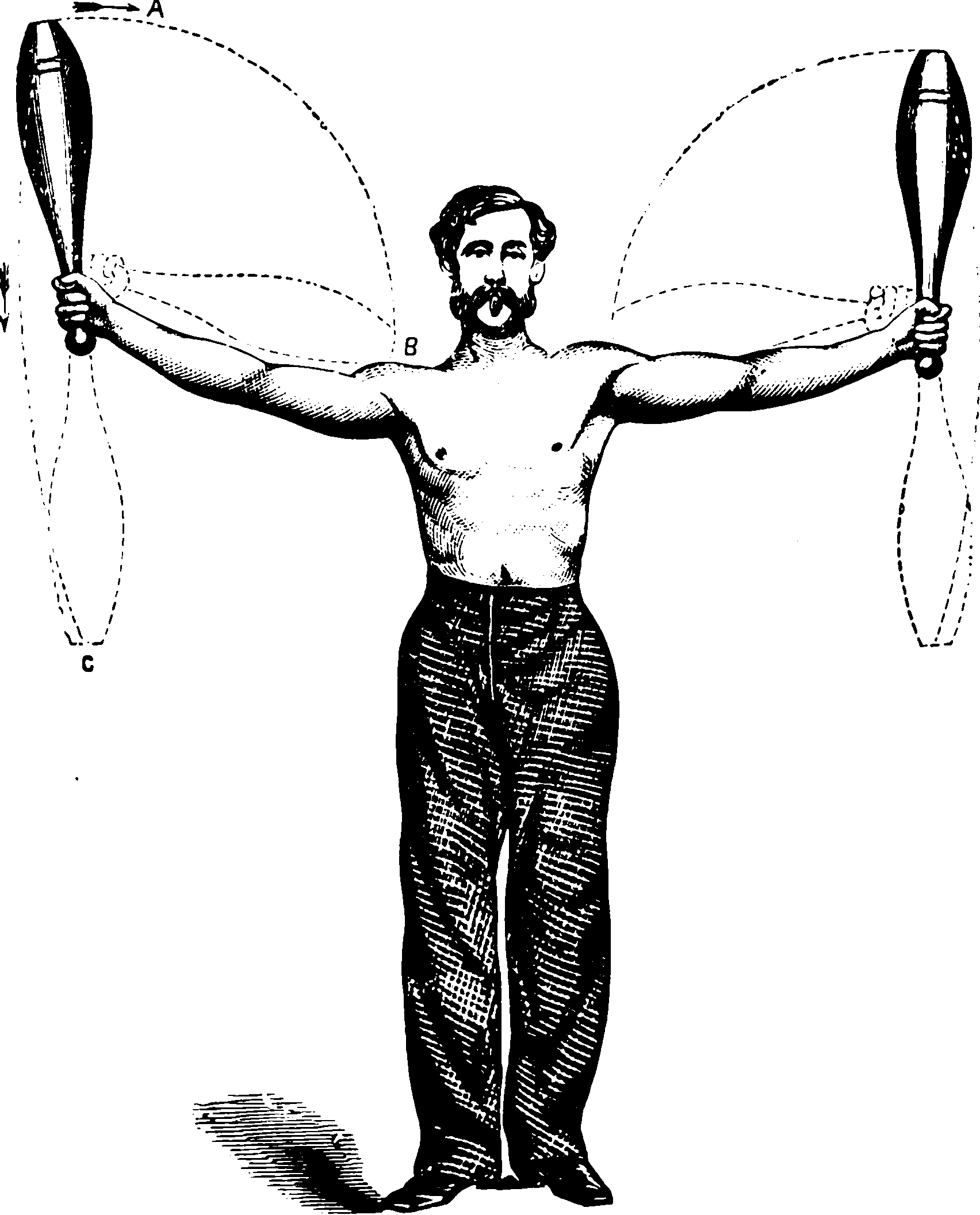# Weighted data in statisticsThomas Lumley helpfully disambiguates the “three and half distinct uses of the term weights in statistical methodology”.

The three main types of weights are

• the ones that show up in the classical theory of weighted least squares. These describe the precision (1/variance) of observations. …. I call these precision weights; Stata calls them analytic weights.
• the ones that show up in categorical data analysis. These describe cell sizes in a data set, so a weight of 10 means that there are 10 identical observations in the dataset, which have been compressed to a covariate pattern plus a count. … Stata calls these frequency weights, and so do I.
• the ones that show up in classical survey sampling theory. These describe how the sample can be scaled up to the population. Classically, they were the reciprocals of sampling probabilities, so an observation with a weight of 10 was sampled with probability 1/10, and represents 10 people in the population. In real life, these are typically more complicated than just sampling probabilities, but they play the same role of trying to rescale the sample distribution to match the population distribution. I call these sampling weights, Stata calls them probability weights, other people call them design weights or grossing-up weights.

The mean formula for each is the same, but not the variance.

TODO: I know that iteratively reweighted least squares fitting is a thing but… why is it not common for other log-additive likelihoods in frequentist statistics? Why do I not have other precision weights for my estimates? We get precision weighting in Bayesian statistics, which looks somewhat similar.

### No comments yet. Why not leave one?

GitHub-flavored Markdown & a sane subset of HTML is supported.